# A variable has a density curve equation y=1 for 0<x<1 and y=0a.graph density curveb. show area under density curve to the left of any number x between 0 + 1 =xc. what percentage of all possibilities of the variable lie between 1/4 and 3/4?d. exceeds 7/8?

Question
4 views

A variable has a density curve equation y=1 for

0<x<1 and y=0

a.graph density curve

b. show area under density curve to the left of any number x between 0 + 1 =x

c. what percentage of all possibilities of the variable lie between 1/4 and 3/4?

d. exceeds 7/8?

check_circle

Step 1

Hey there! Thank you for posting the question. Since your question has more than 3 parts, we are solving the first 3 parts for you, according to our policy. If you need help with any of the other parts, please re-post the question and mention the part you want answered.

Step 2

a)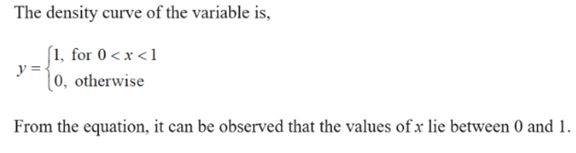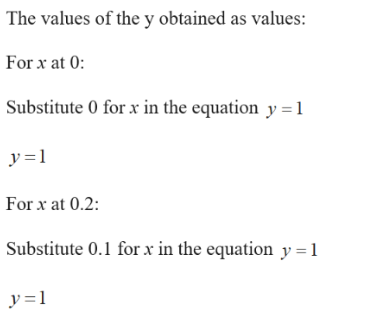Similarly the remaining points are obtained as follows: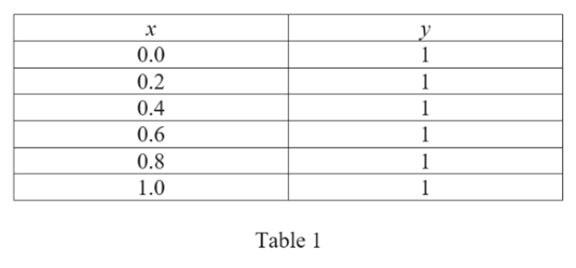Step 3

Procedure for drawing the density curve of the variable y=1 for 0<x<1 otherwise y=0 is as follows:

• Draw the x and y
• Plot the points Table 1, using the horizontal axis for the x values and the vertical axis for the y values as shown below.

The density curve of the given variable is,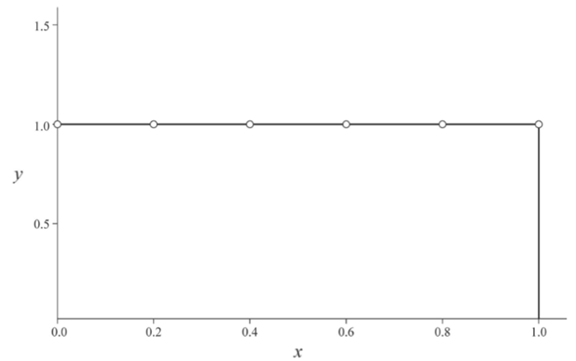(b)

From the given information, it can be observed that the area to the left of any number x between 0 and 1 is the rectangle and the base and height are x and 1.

The area of rectangle is,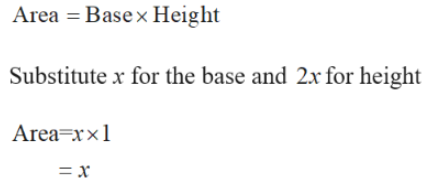...

### Want to see the full answer?

See Solution

#### Want to see this answer and more?

Solutions are written by subject experts who are available 24/7. Questions are typically answered within 1 hour.*

See Solution
*Response times may vary by subject and question.
Tagged in